### SPACECRAFT DEPRESSURISATION

INTRODUCTION

 All manned spacecraft have a life support section that is pressurised with a suitable gas, usually air, to ensure the survival of its occupants. When the integrity of this region is breached, through impact or other cause, the gas escapes from within the spacecraft out into space. The rate of escape is dependent on the size of the breach, the volume of the pressurised space, the initial pressure and the nature of the gas. This note explores the depressurisation time with respect to these parameters.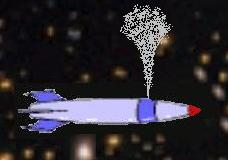BASIC PHYSICS

There are two laws of basic physics that we need to explore the problem of gas escaping through a hole from a pressurised volume into the vacuum of space. One is the ideal gas equation and the other is Bernoulli's law of fluid dynamics.

The Ideal Gas Equation

p V = M R T / μ

where:
p is the pressure of the gas in V
V is the volume which the gas occupies
M is the mass of the gas R is the Universal Gas Constant ( = 8.31 in SI units )
T is the absolute temperature of the gas
μ is the molecular mass of the gas

Bernoulli's Law

p + ½ρv2 + ρgh = constant along a streamline

where:
p is the pressure of the gas
ρ is the density of the gas
v is the velocity of the gas
g is the gravitational acceleration
h is the height above a gravitational datum
Note: In zero gravity the last term is zero.

APPLICATIONThe schematic diagram at left shows the situation and variables to which we shall apply the above laws. The life support volume may be only a fraction of the total spacecraft volume. It is that part of the craft that is pressurised for the sustainability of its crew and any passengers it may carry.

We consider a streamline that passes through the hole, and apply Bernoulli's law to a point just inside the hole and second point just outside the hole. We assume that in this short distance the gas density remains constant. This gives us the equation:

pi + ½ρvi2 = po + ½ρve2

Noting that the pressure in space (po) is essentially zero, and that the gas velocity (vi) inside the spacecraft is a lot less than the escape velocity (ve) of the gas outside the craft (and thus can be neglected with respect to it), we have that:

pi = ½ρve2

giving an expression for the escape velocity of:

ve = √( 2 pi / ρ )

We can consider the volume of gas escaping each second as having a cylindrical volume with a diameter equal to the diameter of the hole and a length equal to the distance travelled by the gas in one second. The mass of the gas escaping in one second is then this volume times the gas density. This is the rate of mass loss per unit time, and may be written as:

dm/dt = ρ Ah ve

Substituting in the previous expression for exhaust velocity we find:

dm/dt = Ah √(2 pi ρ ) ...[eq.1]

We note that density ρ = M / V ...[eq.2]

and we use the Ideal Gas Equation to find the relation between pi and ρ :

pi = ρ R T / μ ...[eq.3]

We can now use the last three numbered equations to iteratively solve for the time variation of cabin pressure, mass and/or density.

IMPLEMENTATION

An algorithm to calculate the time variation of cabin atmospheric parameters is specified as follows:

• Step 1 - Specify initial pressure
• Step 2 - Compute initial density (eq.3), inital mass (eq.2)
• Step 3 - Compute mass loss per unit time (eq.1)
• Step 4 - Compute new mass at new time (Mnew = Mold - massloss)
• Step 5 - Compute new density (eq.2), new pressure (eq.3)
• Step 6 - Repeat from step 3 as required

The following table contains the code for a simple program in Quick Basic that implements the above algorithm, displaying a table of gas pressure, mass and density every 100 seconds.

 ```'DEPRESSURATION TIME FOR HOLED SPACECRAFT CLS 'clear screen mw = .029 'molecular weight of gas (kg/mole) - air=0.029 temp = 293 'temp in kelvin (=20 Celcius) Rg = 8.314 'Universal Gas Constant (J / mole-K) press = 100000 'pressure in Pascal (Earth atmospheric = 101,300 Pa) press0 = press 'keep record of initial pressure vol = 30 'spacecraft cabin volume cubic metres Ah = .0001 'impact hole in square metres tm = 0 'start time (seconds) PRINT USING "Spacecraft depressurisation - Hole size = #####.## sq cm"; Ah * 10000 PRINT "Time(sec/min) Mass(kg) Density(kg/m^3) Pressure(kPa)" f1\$ = " ####/###.# ###.# ##.## ###.##" 'compute initial mass and density of gas mass = press * vol * mw / Rg / temp 'initial mass of gas rho = mass / vol 'initial density 'now advance in time DO 'print out parameters every 100 seconds IF tm MOD 100 = 0 THEN PRINT USING f1\$; tm; tm / 60; mass; rho; press / 1000 END IF tm = tm + 1 'advance time by one second massloss = Ah * SQR(2 * press * rho) 'compute mass loss in 1 sec mass = mass - massloss 'compute new mass rho = mass / vol 'compute new density press = rho * Rg * temp / mw .compute new pressure LOOP WHILE press > press0 / 10 'do while pressure>10% initial ```

The next table presents the output of the above program with the parameters as specified.

 ``` Spacecraft depressurisation - Hole size = 1.00 sq cm Time(sec/min) Mass(kg) Density(kg/m^3) Pressure(kPa) 0/ 0.0 35.7 1.19 100.00 100/ 1.7 31.2 1.04 87.22 200/ 3.3 27.2 0.91 76.08 300/ 5.0 23.7 0.79 66.35 400/ 6.7 20.7 0.69 57.88 500/ 8.3 18.0 0.60 50.48 600/ 10.0 15.7 0.52 44.03 700/ 11.7 13.7 0.46 38.40 800/ 13.3 12.0 0.40 33.50 900/ 15.0 10.4 0.35 29.22 1000/ 16.7 9.1 0.30 25.48 1100/ 18.3 7.9 0.26 22.23 1200/ 20.0 6.9 0.23 19.39 1300/ 21.7 6.0 0.20 16.91 1400/ 23.3 5.3 0.18 14.75 1500/ 25.0 4.6 0.15 12.86 1600/ 26.7 4.0 0.13 11.22 ```

RESULTS

The following graph shows how cabin pressure decreases with time. The different curves indicate different hole sizes (in terms of the hole area). In these graphs the cabin volume is assumed to be 30 cubic metres and the initial pressure 100 kilopascals of air (20% oxygen and 80% nitrogen). The mean atmospheric pressure on the Earth's surface is 101.3 kPa.

We can see that a one square centimetre hole will reduce cabin pressure by 50% in 500 seconds (8.3 minutes). This value scales in inverse proportion to hole area. Thus a 10 sq cm hole will only take 50 seconds to halve the pressure, whereas a 0.1 sq cm hole (10 square millimetres) will take 5000 seconds.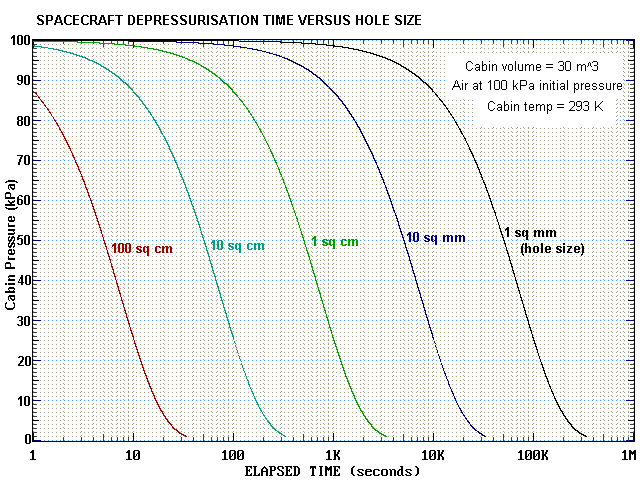The next graph assumes a hole of one square centimetre and plots how the cabin pressure varies with time for a range of cabin volumes. The smallest volume (3 m3) might correspond to a personal escape pod, the next (30 m3) to a multiperson reentry capsule, and the last two to small and large space stations respectively.

In this case the time to reduce the pressure by half scales linearly with the cabin size. Thus while a 30 cubic metre cabin will depressurise by 50% in 500 sceonds, a 300 cubic metre space station will take 5000 seconds for a 50% reduction in pressure.Now we show how different gases and initial pressures affect the depressurisation time. Again we assume a fixed hole size of one square centimetre. Gases with a higher molecular weight than air will show a longer time to depressurise and gases with a lower molecular weight a shorter time. Pure oxygen (MW=0.032 kg/mole) takes slighly longer to leave the cabin than does air (MW=0.029). A helium-oxygen mix (MW=0.0.10) takes less time. The depressurisation time scales linearly with the gas molecular weight.

If pure oxygen was used as a cabin atmosphere it would normally be present at a lower initial pressure anyway. At an inital pressure of 20 kPa (which corresponds to the partial pressure it has in 100 kPa air) the 50% depressurisation time is identical to that of pure oxygen at 100 kPa. Thus we see that initial pressure does not affect the depressurisation time.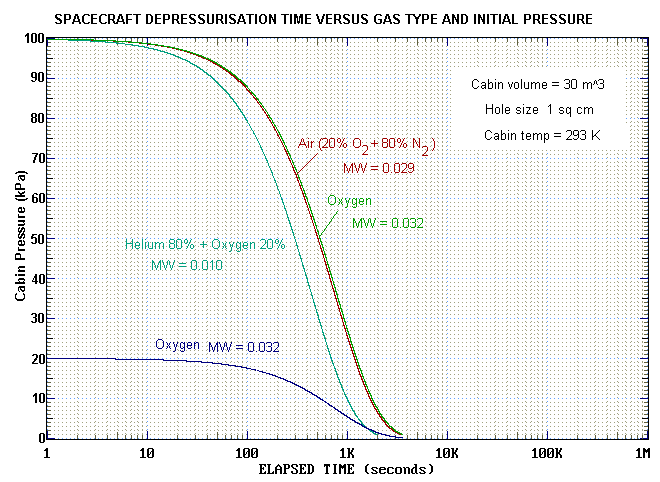All three graphs above have assumed that the depressurisation process is isothermal (constant temeperature) with a cabin temperature of 293 K. This is a reasonably valid assumption as the cabin and surroundings will have a much greater thermal mass than the air within. The specified temperature will also be close to that necessary to keep humans comfortable. However, it is interesting to investigate how temperature affects the time to depressure. The following graph plots time versus cabin pressure for temperatures of 293 K (20 C) and 29 K (-244 C). This latter value might be the temperature of a derelict craft that has been abandoned in the outer solar system for some time before suffering an impact that produces a 1 sq cm hole.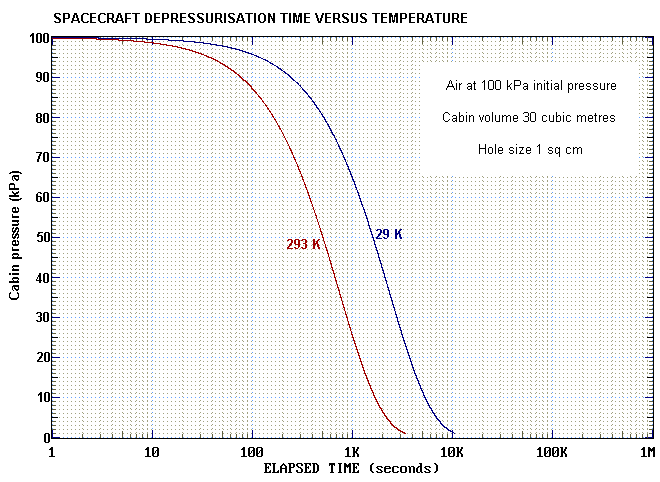Two more factors must be mentioned that may affect the depressurisation time. First we note that a gas cannot expand at a velocity greater than its speed of sound. This may in some cases decrease the rate at which gas escapes and thus lengthen the depressurisation time. Secondly, if the hole size is smaller than the wall thickness there will be a resistance to flow through the resultant non-zero length pipe due to a slower boundary layer at the edges of the hole. This will also serve to limit the escape rate and increase the time to depressure. The times shown in the graphs above should thus be regarded as minimum times.

SIGNIFICANCE

Most humans live in an atmosphere of 20% oxygen and 80% inert gas (mostly nitrogen) at a total pressure of around 100 kilopascal (kPa). The nominal pressure at sea-level on the Earth is 101.3 kPa and this decreases with altitude by roughly ten kPa for every kilometre, at least for the first few kilometres.

For a person accustomed to living on Earth at sea-level the first symptoms of hypoxia (lack of oxygen) appear around an altitude of 3000 m. The visual system at low light levels is impaired (pilots of light aircraft without supplemental oxygen are advised not to fly above 3000 m (10,000 feet) at night). Breathlessness will occur under exertion, and it may be more difficult to sleep. On the island of Hawaii there are many large optical telescopes on the peak of Mauna Kea, which is 4,200 m high. Astronomers who work on this mountain do not spend large amounts of time at the peak, but are accommodated at a lower level around 2,700 m. There is also a visitor's centre at this height, and anyone who wants to ascend to the summit to see the telescopes and do a little stargazing is advised to spend a few hours at this level to acclimatise before going on to the peak.

It is of course, possible to acclimatise to high altitudes, and small populations of people do live above 3,000 m. People are also diverse and different people experience hypoxia at different partial pressures of oxygen.

However, as a rule of thumb, a person accustomed to breathing air at sea-level pressure (100 kPa) tends to lose consciousness at about 30 kPa, and the ability to perform useful work will occur before this pressure is reached.

If we use a value of 30 kPa as the lowest pressure of air that can possibly sustain human consciousness, and note that this pressure is reached in about 30 seconds when a cabin of one cubic metre pressurised to 100 kPa is breached by a 1 square centimetre hole, we can use the scaling rules noted above to give a quick formula:

Lifetime(secs) = 30 * Cabin_Volume(m3) / Hole_Size(cm2)

Once again we note that this is a minimum time. Other factors may literally provide more breathing time, and of course a person does not die immediately after losing consciousness. However, if no remedial action occurs (eg from an outside source or another astronaut in a space suit), this time is effectively the useful lifetime of the individual.

HISTORICAL

 As of mid-2011 the only incident involving spacecraft depressurisation that resulted in fatalities was that of the returning Soyuz 11 capsule in 1971. This tragic incident was not due to a hypervelocity impact but is believed to have occurred because a ventilation valve was jolted open when the descent module separated from the service module at the start of reentry back to Earth. The three cosmonauts were found dead inside the capsule when it was opened after landing.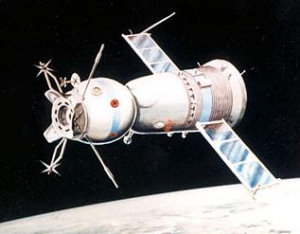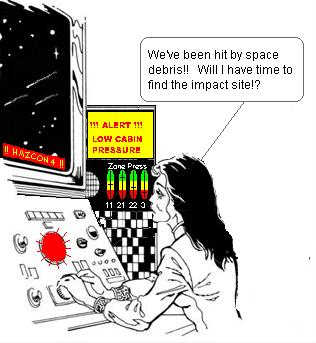Australian Space Academy